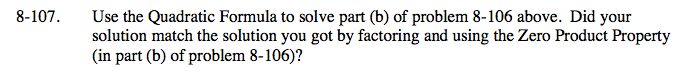### Home > CAAC > Chapter 8 > Lesson 8.3.2 > Problem8-107

8-107.$\frac{-b\pm\sqrt{b^{2}-4ac}}{2a}$

3x2 + 17x − 6 = 0

a = 3 b = 17 c = −6

$x=\frac{-17\pm\sqrt{17^{ \; 2}-4(3)(-6)}}{2(3)}$

Solve the equation and compare your answer to part (b) of problem 8-106.